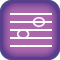Intervals III: Sixths and Sevenths

Skills covered:
 Major and Minor SixthsMajor and Minor Sevenths Spelling IntervalsRecognizing Intervals by Ear

1.  Major and minor sevenths
Lesson

2.  Major and minor sixths
Lesson

3.  Aural recognition of m2, M2, m6 and M6 (harmonic)
Lesson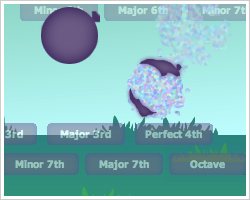Harmonic Drops
Level 11

4.  m2, M2, m6 and M6 in major keys (solfege)
Lesson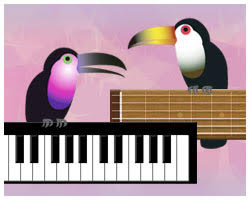Two Tones (Major)
Level 11

5.  m2, M2, m6 and M6 in minor keys (solfege)
Lesson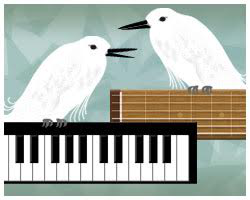Two Tones (Minor)
Level 11

6.  Notation of m2, M2, m6 and M6
Lesson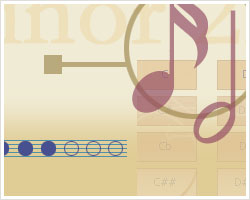Flash Notation (Intervals)
Level 11

7.  Aural recognition of P4, d5, P5, m6 and M6 (melodic)
Lesson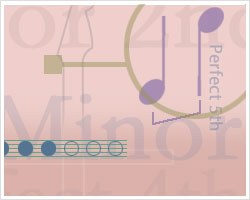Flash Intervals (Mel.)
Level 11

8.  Aural recognition of P4, d5, P5, m6 and M6 (harmonic)
Lesson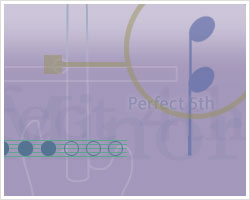Flash Intervals (Harmonic)
Level 11

9.  Aural recognition of m2, M2, m6 and M6 (melodic)
Lesson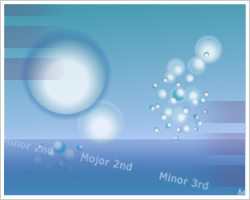Melodic Drops
Level 11

10.  Aural recognition of P4, d5, P5, m7 and M7 (melodic)
LessonFlash Intervals (Mel.)
Level 12

11.  Aural recognition of P4, d5, P5, m7 and M7 (harmonic)
LessonFlash Intervals (Harmonic)
Level 12

12.  Aural recognition of m3, M3, m7 and M7 (melodic)
LessonMelodic Drops
Level 12

13.  Notation of m3, M3, m7 and M7
LessonFlash Notation (Intervals)
Level 12

14.  m3, M3, m7 and M7 in minor keys (solfege)
LessonTwo Tones (Minor)
Level 12

15.  m3, M3, m7 and M7 in major keys (solfege)
LessonTwo Tones (Major)
Level 12

16.  Aural recognition of m3, M3, m7 and M7 (harmonic)
LessonHarmonic Drops
Level 12

17.  Aural recognition of P4, d5, P5, m6, M6, m7 and M7 (harmonic)
LessonFlash Intervals (Harmonic)
Level 13

18.  Aural recognition of P4, d5, P5, m6, M6, m7 and M7 (melodic)
LessonFlash Intervals (Mel.)
Level 13

19.  m2, M2, m6, M6, m7 and M7 in minor keys (solfege)
LessonTwo Tones (Minor)
Level 13

20.  Notation of m2, M2, m6, M6, m7 and M7
LessonFlash Notation (Intervals)
Level 13

21.  Aural recognition of m2, M2, m6, M6, m7 and M7 (harmonic)
LessonHarmonic Drops
Level 13

22.  Aural recognition of m2, M2, m6, M6, m7 and M7 (melodic)
LessonMelodic Drops
Level 13

23.  m2, M2, m6, M6, m7 and M7 in major keys (solfege)
LessonTwo Tones (Major)
Level 13

24.  Notation of m3, M3, m6, M6, m7 and M7
LessonFlash Notation (Intervals)
Level 14

25.  Aural recognition of m3, M3, P4, d5, P5, m6, M6, m7 and M7 (melodic)
LessonFlash Intervals (Mel.)
Level 14

26.  Aural recognition of m3, M3, P4, d5, P5, m6, M6, m7 and M7 (harmonic)
LessonFlash Intervals (Harmonic)
Level 14

27.  m3, M3, m6, M6, m7 and M7 in minor keys (solfege)
LessonTwo Tones (Minor)
Level 14

28.  m3, M3, m6, M6, m7 and M7 in major keys (solfege)
LessonTwo Tones (Major)
Level 14

29.  Aural recognition of m3, M3, m6, M6, m7 and M7 (harmonic)
LessonHarmonic Drops
Level 14

30.  Aural recognition of m3, M3, m6, M6, m7 and M7 (melodic)
LessonMelodic Drops
Level 14

31.  Aural recognition of m2, M2, m3, M3, m6, M6, m7 and M7 (melodic)
LessonMelodic Drops
Level 15

32.  Aural recognition of m2, M2, m3, M3, m6, M6, m7 and M7 (harmonic)
LessonHarmonic Drops
Level 15

33.  m2, M2, m3, M3, m6, M6, m7 and M7 in major keys (solfege)
LessonTwo Tones (Major)
Level 15

34.  m2, M2, m3, M3, m6, M6, m7 and M7 in minor keys (solfege)
LessonTwo Tones (Minor)
Level 15

35.  Notation of m2, M2, m3, M3, m6, M6, m7 and M7
LessonFlash Notation (Intervals)
Level 15

36.  Aural recognition of m2, M2, m3, M3, P4, d5, P5, m6, M6, m7 and M7 (melodic)
LessonFlash Intervals (Mel.)
Level 15

37.  Aural recognition of m2, M2, m3, M3, P4, d5, P5, m6, M6, m7 and M7 (harmonic)
LessonFlash Intervals (Harmonic)
Level 15